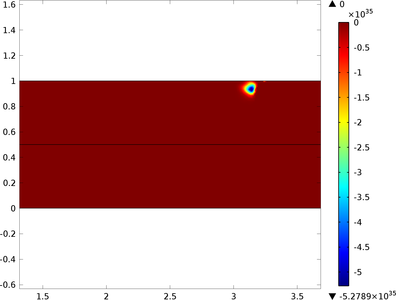# Galleria delle Applicazioni

## Breakdown in a MOSFET

Application ID: 15583

MOSFETs typically operate in three regimes depending on the drain-source voltage for a given gate voltage. Initially the current-voltage relation is linear, this is the Ohmic region. As the drain-source voltage increases the extracted current begins to saturate, this is the saturation region. As the drain-source voltage is further increased the breakdown region is entered, where the current increases exponentially for a small increase in the applied voltage. This is due to impact ionization.

This model shows how to use the time dependent solver to model impact ionization in a MOSFET.This model example illustrates applications of this type that would nominally be built using the following products: